# SimBiology.gsa.MPGSA

Object containing multiparametric global sensitivity analysis (MPGSA) results

## Description

The `SimBiology.gsa.MPGSA` object contains multiparametric global sensitivity analysis  results returned by `sbiompgsa`.

## Creation

Create a `SimBiology.gsa.MPGSA` object using `sbiompgsa`.

## Properties

expand all

Expressions of model responses, specified as a cell array of character vectors.

Data Types: `cell`

Kolmogorov-Smirnov statistics, specified as a table. The table size is [params,classifiers], where params is the number of input parameters and classifiers is the number of classifiers. Entry `[i,j]` contains the Kolmogorov-Smirnov statistic returned by `kstest2` (Statistics and Machine Learning Toolbox) comparing the two eCDFs of the ith parameter accepted and rejected by the jth classifier. If all samples are accepted or rejected by the classifier, entry `[i,j]` is set to `NaN`.

The `VariableNames` property contains the classifier expressions specified as the input to `sbiompgsa`. Long expressions are truncated with the addition of `'(classifier i)'`, where i is the classifier index. The `VariableDescriptions` property contains the untruncated classifier expressions.

Data Types: `table`

Computed eCDF data, specified as a cell array of numeric vectors. The size of the array is `[params,4,classifiers]`, where params is the number of input parameters and classifiers is the number of classifiers.

Cells `[i,1:2,j]` contain the f (Statistics and Machine Learning Toolbox) and x (Statistics and Machine Learning Toolbox) outputs from the `ecdf` (Statistics and Machine Learning Toolbox) function for the samples of parameter i accepted by the classifier j.

Cells `[i,3:4,j]` contain the corresponding outputs for the samples of parameter i rejected by the classifier j.

If the classifier accepts all samples or rejects all samples, the corresponding eCDF data is empty.

Data Types: `cell`

Significance level of the two-sided Kolmogorov-Smirnov test, specified as a scalar value in the range (0,1). For details, see `kstest2` (Statistics and Machine Learning Toolbox) .

Data Types: `double`

Asymptotic p-values for the Kolmogorov-Smirnov tests, specified as a table. The table size is [params,classifiers], where params is the number of input parameters and classifiers is the number of classifiers.

Entry `[i,j]` contains the p-values returned by `kstest2` (Statistics and Machine Learning Toolbox) comparing the two eCDFs of the ith parameter being accepted and rejected by the jth classifier. If all samples are accepted or rejected by the classifier, entry `[i,j]` is set to `NaN`.

The `VariableNames` property contains the classifier expressions specified as the input to `sbiompgsa`. Long expressions are truncated with the addition of `'(classifier i)'`, where i is the classifier index. The `VariableDescriptions` property contains the untruncated classifier expressions.

Data Types: `table`

Flags indicating if the samples are accepted by the classifiers, specified as a table. The table size is [NumberSamples,classifiers], where NumberSamples is the number of parameter samples and classifiers is the number of classifiers.

The `VariableNames` property contains the classifier expressions specified as the input to `sbiompgsa`. Long expressions are truncated with the addition of `'(classifier i)'`, where i is the classifier index. The `VariableDescriptions` property contains the untruncated classifier expressions.

Data Types: `table`

Names of model responses or observables, specified as a cell array of character vectors.

Data Types: `char`

Sampled parameter values, specified as a table. Each row represents one parameter set and each column represents one input parameter. For details, see Multiparametric Global Sensitivity Analysis (MPGSA).

Data Types: `table`

Simulation information, such as simulation data and parameter samples, used for multiparametric global sensitivity analysis, specified as a structure. The structure contains the following fields.

• `SimFunction``SimFunction` object used for simulating model responses or observables.

• `SimData``SimData` array of size `[NumberSamples,1]`, where NumberSamples is the number of samples. The array contains simulation results from `ParameterSamples`.

• `OutputTimes` — Numeric column vector containing the common time vector of all `SimData` objects.

• `Bounds` — Numeric matrix of size `[params,2]`. params is the number of input parameters. The first column contains the lower bounds and the second column contains the upper bounds for sensitivity inputs.

This field is set to `[]` if you provided parameter sample values as input when you called `sbiompgsa`.

• `DoseTables` — Cell array of dose tables used for the `SimFunction` evaluation. `DoseTables` is the output of `getTable(doseInput)`, where doseInput is the value specified for the `'Doses'` name-value pair argument in the call to `sbiosobol`, `sbiompgsa`, or `sbioelementaryeffects`. If no doses are applied, this field is set to `[]`.

• `ValidSample` — Logical vector of size `[NumberSamples,1]` indicating whether a simulation for a particular sample failed. Resampling of the simulation data (SimData) can result in `NaN` values if the data is extrapolated. Such SimData are indicated as invalid.

• `InterpolationMethod` — Name of the interpolation method for `SimData`.

• `SamplingMethod` — Name of the sampling method used to draw `ParameterSamples`. When you call `sbiompgsa` with `samples` (sampled model quantities) as the input, this field of the corresponding results object is set to `'unknown'`.

• `RandomState` — Structure containing the state of `rng` before drawing `ParameterSamples`. When you call `sbiompgsa` with `samples` (parameter sample values) as an input, this property of the corresponding results object is `[]`.

Data Types: `struct`

## Object Functions

 `plotData` Plot quantile summary of model simulations from global sensitivity analysis (requires Statistics and Machine Learning Toolbox) `plot` Plot empirical CDF of multiparametric global sensitivity analysis `bar` Create bar plot of multiparametric global sensitivity analysis statistics `histogram` Plot histogram of multiparametric global sensitivity analysis results

## Examples

collapse all

`sbioloadproject tmdd_with_TO.sbproj`

Get the active configset and set the target occupancy (`TO`) as the response.

```cs = getconfigset(m1); cs.RuntimeOptions.StatesToLog = 'TO';```

Simulate the model and plot the `TO` profile.

`sbioplot(sbiosimulate(m1,cs));`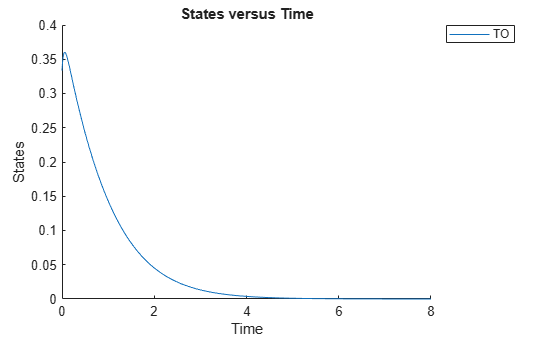Define an exposure (area under the curve of the TO profile) threshold for the target occupancy.

`classifier = 'trapz(time,TO) <= 0.1';`

Perform MPGSA to find sensitive parameters with respect to the TO. Vary the parameter values between predefined bounds to generate 10,000 parameter samples.

```% Suppress an information warning that is issued during simulation. warnSettings = warning('off', 'SimBiology:sbservices:SB_DIMANALYSISNOTDONE_MATLABFCN_UCON'); rng(0,'twister'); % For reproducibility params = {'kel','ksyn','kdeg','km'}; bounds = [0.1, 1; 0.1, 1; 0.1, 1; 0.1, 1]; mpgsaResults = sbiompgsa(m1,params,classifier,Bounds=bounds,NumberSamples=10000)```
```mpgsaResults = MPGSA with properties: Classifiers: {'trapz(time,TO) <= 0.1'} KolmogorovSmirnovStatistics: [4x1 table] ECDFData: {4x4 cell} SignificanceLevel: 0.0500 PValues: [4x1 table] SupportHypothesis: [10000x1 table] ParameterSamples: [10000x4 table] Observables: {'TO'} SimulationInfo: [1x1 struct] ```

Plot the quantiles of the simulated model response.

`plotData(mpgsaResults,ShowMedian=true,ShowMean=false);`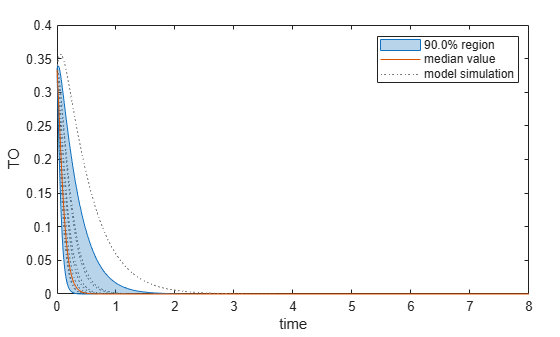Plot the empirical cumulative distribution functions (eCDFs) of the accepted and rejected samples. Except for `km`, none of the parameters shows a significant difference in the eCDFs for the accepted and rejected samples. The `km` plot shows a large Kolmogorov-Smirnov (K-S) distance between the eCDFs of the accepted and rejected samples. The K-S distance is the maximum absolute distance between two eCDFs curves.

```h = plot(mpgsaResults); % Resize the figure. pos = h.Position(:); h.Position(:) = [pos(1) pos(2) pos(3)*2 pos(4)*2];```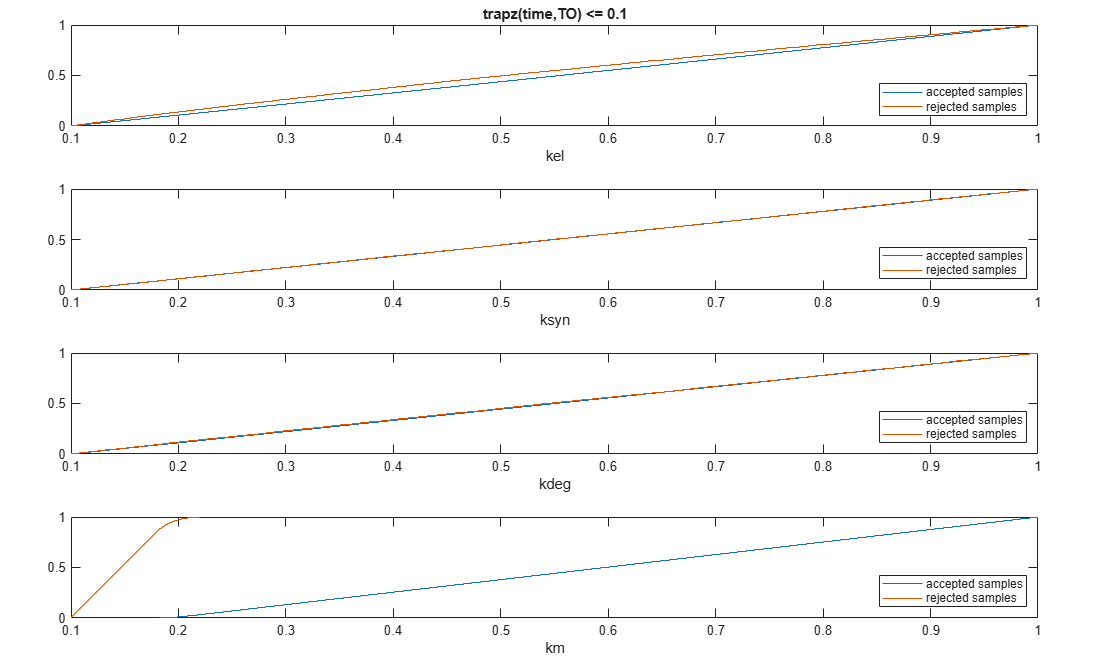To compute the K-S distance between the two eCDFs, SimBiology uses a two-sided test based on the null hypothesis that the two distributions of accepted and rejected samples are equal. See `kstest2` (Statistics and Machine Learning Toolbox) for details. If the K-S distance is large, then the two distributions are different, meaning that the classification of the samples is sensitive to variations in the input parameter. On the other hand, if the K-S distance is small, then variations in the input parameter do not affect the classification of samples. The results suggest that the classification is insensitive to the input parameter. To assess the significance of the K-S statistic rejecting the null-hypothesis, you can examine the p-values.

`bar(mpgsaResults)`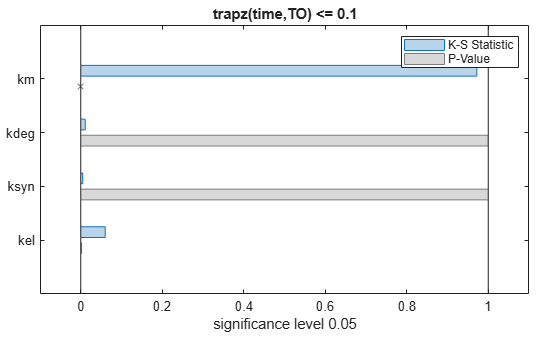The bar plot shows two bars for each parameter: one for the K-S distance (K-S statistic) and another for the corresponding p-value. You reject the null hypothesis if the p-value is less than the significance level. A cross (`x`) is shown for any p-value that is almost 0. You can see the exact p-value corresponding to each parameter.

`[mpgsaResults.ParameterSamples.Properties.VariableNames',mpgsaResults.PValues]`
```ans=4×2 table Var1 trapz(time,TO) <= 0.1 ________ _____________________ {'kel' } 0.0021877 {'ksyn'} 1 {'kdeg'} 0.99983 {'km' } 0 ```

The p-values of `km` and `kel` are less than the significance level (0.05), supporting the alternative hypothesis that the accepted and rejected samples come from different distributions. In other words, the classification of the samples is sensitive to `km` and `kel` but not to other parameters (`kdeg` and `ksyn`).

You can also plot the histograms of accepted and rejected samples. The historgrams let you see trends in the accepted and rejected samples. In this example, the histogram of `km` shows that there are more accepted samples for larger `km` values, while the `kel` histogram shows that there are fewer rejected samples as `kel` increases.

```h2 = histogram(mpgsaResults); % Resize the figure. pos = h2.Position(:); h2.Position(:) = [pos(1) pos(2) pos(3)*2 pos(4)*2];```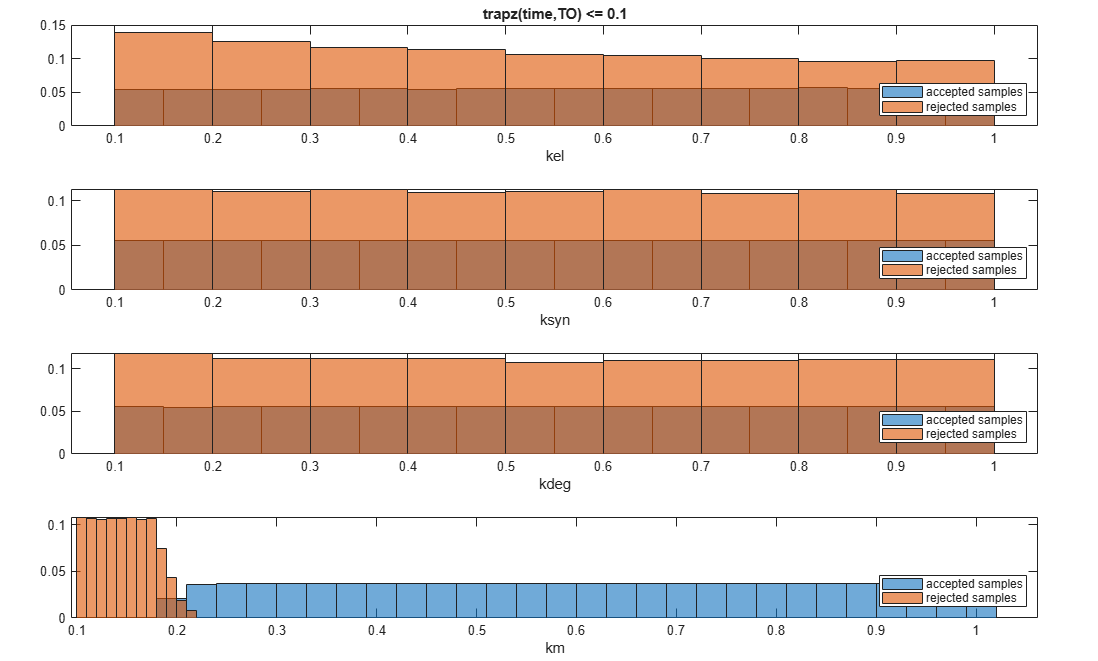Restore the warning settings.

`warning(warnSettings);`